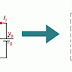Thevenin’s theorem states that a linear and bilateral network can be replaced by an equivalent circuit consisting of a voltage source Vth in series with a resistance Rth.
In any linear bilateral network having more than one element, it can be replaced by a single equivalent circuit consisting of an equivalent voltage source (Vth) in series with equivalent resistance (Rth).

Thevenin’s equivalent circuit resembles a practical voltage source. Hence, it has a voltage source in series with a resistance.
• The voltage source present in the Thevenin’s equivalent circuit is called as Thevenin’s equivalent voltage or Thevenin’s voltage, Vth.
• The resistor present in the Thevenin’s equivalent circuit is called as Thevenin’s equivalent resistor or simply Thevenin’s resistance Rth.

### Conclusion:-

Thevenin Theorem is a basic equivalence principle for circuit design. It can simplify a very complex circuit into a very simple equivalent. This is done by finding the Thevenin Resistance (Rth) as well as the Thevenin voltage (Vth) and current. Once they are known, the equivalent circuit (or network) is simply a voltage source in series with a resistance.
• Thevenin’s Theorem is a way to reduce a network to the equivalent circuit (or network) composed of a single voltage source, series resistance, and series load.
• Steps to follow for Thevenin’s Theorem:-
• Find the Thevenin source voltage by removing the load resistance (RL) from the original circuit and calculating the voltage across the open connection points where the load resistor used to be.
• Find the Thevenin resistance (Rth) by removing all power sources in the original circuit (voltage sources shorted (S.C) and current sources open(O.C)) and calculating total resistance between the open connection points.
• Draw the Thevenin equivalent circuit, with the Thevenin voltage source (Vth) in series with the Thevenin resistance( Rth). The load resistance (RL) re-attaches between the two open points of the equivalent circuit.
• Analyze voltage and current for the load resistance (RL) following the rules for series circuits.

### Reference:-

Thevenin’s TheoremReviewed by FacebookHint on January 19, 2020 Rating: 5